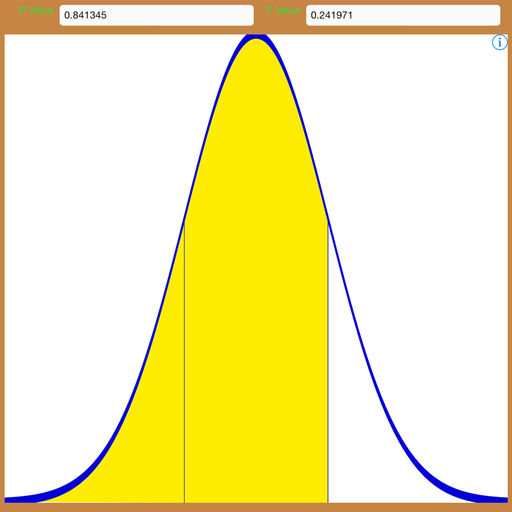Scientific Calculator With Normal/Gaussian Distribution# Scientific Calculator With Normal/Gaussian Distribution

## MODULAR Software & Services GmbH

2.0 for iPhone, iPad
Free
0

0 Ratings

Release Date

2014-11-05

Size

2.8 MB

### Screenshots for iPhone

iPhone
More Screenshots for iPad
Scientific Calculator With Normal/Gaussian Distribution Description
F-Graph calculates and plots the standard normal distribution frequency and density functions.

One tail, middle, two tail and cumulative density functions (probabilities) can be calculated together with the raw frequency function.

You may enter directly Z values referred to N(0,1), or X values referred to a generic N(m, s).

In case of X values, the values for m=mean and s=standard deviation are also required. If Z values are entered, X values are computed according to m and s and the other way around.

F-Graph also works as a scientific calculator. You may enter the calculation clicking on the buttons of the application pad or directly directly enter the expression as text. Press = to perform the calculation. The calculator supports multiple level of parentheses. A parenthesis is automatically appended when a function button is pressed. You will have then to enter the argument and the closing brace.
Supported functions:
ln(X) logarithm base e
log(X) logarithm base 10
log2 logarithm base 2
exp(X) e to X
rtod radians to decimal degrees
dtor decimal dgress to radians
sqrt(X) square root of X
nthroot(X, N) Nth root of X
pow(N, X) N to X
all common trigonometric functions. Arguments are entered and returned in radians
Scientific Calculator With Normal/Gaussian Distribution 2.0 Update
2014-11-05 Version History
Improved UI
Added general purpose scientific calculator
Support of iOS 8
More